In this article, I will be sharing how to predict the sentiment of financial news using a transformer model, which achieves remarkable accuracy scores. Since there are no good and accessible pre-trained models for financial news out, I had to train one myself. Predicting the sentiment (neutral, positive, negative) of a financial news article can be tremendously useful when making sense of price shifts in the market or figuring out how certain stocks and assets are correlated.

## Data

For the explanatory purpose of this article, we will be using the FinancialPhraseBank which contains 4837 Financial News, each having a sentiment class assigned (neutral, positive, negative). The dataset has been released with the research paper Good Debt or Bad Debt: Detecting Semantic Orientations in Economic Texts  which aimed at generating a human-annotated finance phrase-bank, to use as a benchmark for alternative models. A short extract of the dataset can be seen below.

The authors of the paper released different versions of the dataset which vary based on classification labels for each news sequence. The defined labels can change depending on how many annotators agreed on a classification for a specific news sequence. Hence, we can choose from the 50%, 66%, 75%, and 100% agreed-on dataset versions. For our purposes, we choose the 100% agreed-on defined labels to have a training set which does not suffer from ambiguity. Thus, the amount of news records decreases from 4837 to 2364.

By plotting the distribution of the word and character lengths of each news sequence we obtain a better understanding of your data. The minimum and the maximum number of characters per sequence are 0 and 315, respectively. For the number of words per sequence, the distribution looks similar and has min & max words of 0 and 81, respectively.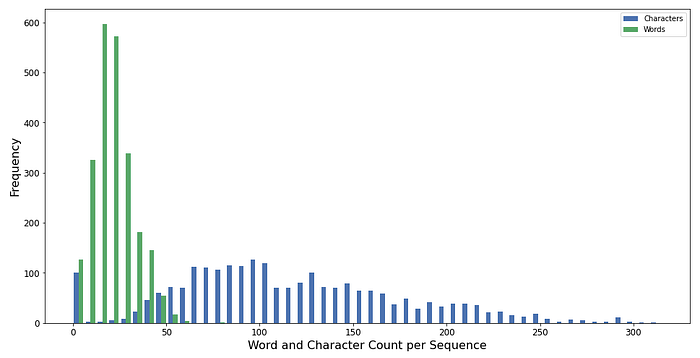Source: FinancePhraseBank word and character count distribution — Created by author

### Data preparation

Since we can’t feed words into our transformer, we have to factorize the sentiment labels. Meaning, that the sentiment neutral is equivalent to 0, positive is 1, and negative is equal to 2.

``df['sentiment_id'] = pd.factorize(df['sentiment'])``

After having assigned a new label number to each news record the dataset looks as follows.

The last step which is needed to prepare our data for the model ingestion is to split the overall dataset into an 80/20, training and validation set. Resulting in a training dataset that has 1891, and a validation dataset which has 473 records.

``````df_train = df.sample(frac=0.8, random_state=3, replace=False)
df_val = df.drop(df_train.index)``````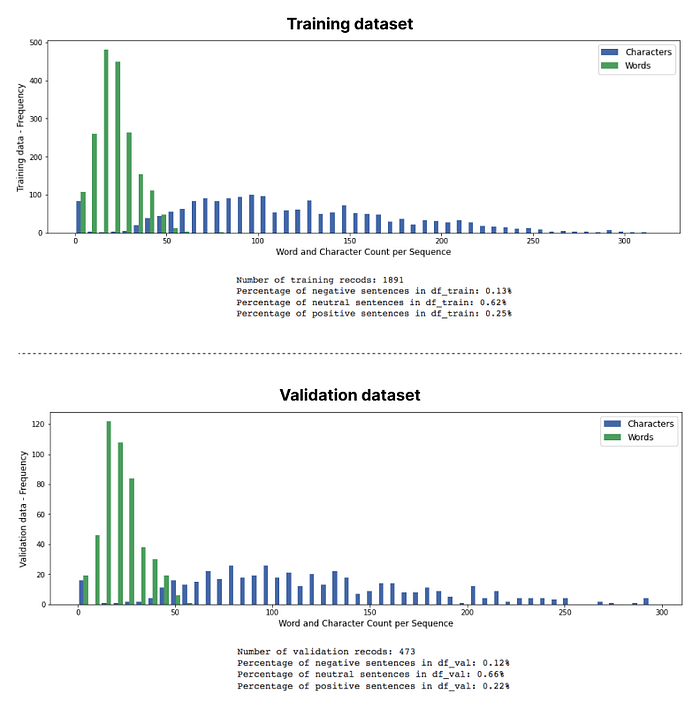Source: Training and validation set word and character count distribution — Created by author

Before we can start implementing and fine-tuning our transformer we first have to calculate the weight of each class in the training dataset and reverse them. These weights have to be parsed to the network during the training process, to avoid the model converging to the benefit of the larger classes. The reversed weights for each class are calculated with the formulas below.

``````weight_for_0 = (1/df_train.loc[df['sentiment'] == 'neutral', 'sentiment'].count())*len(df_train)/3
weight_for_1 = (1/df_train.loc[df['sentiment'] == 'positive', 'sentiment'].count())*len(df_train)/3
weight_for_2 = (1/df_train.loc[df['sentiment'] == 'negative', 'sentiment'].count())*len(df_train)/3
CLASS_WEIGHTS = {0: weight_for_0, 1: weight_for_1, 2: weight_for_2}``````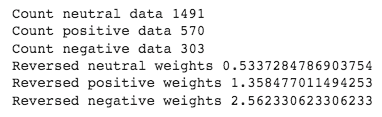Source: Classe count in training dataset and reverse class weight— Created by author

## Transformer

In order to obtain the best sentiment prediction possible, the best approach is to fine-tune a generic and existing language model, instead of training a network from scratch with only our FinancialPhraseBank data which we have at hand.

Hence the next question arises, what language model do we select for fine-tuning? In a previous article, I shared a detailed comparison of language models and how they perform compared to the number of parameters each model has (all of them are transformer networks).

The column Model Performance/Size Ration shows that the ALBERT Transformer models provide the best performance per parameter. This is due to their internal architecture which enables sharing of parameters between each transformer layer. By selecting an ALBERT model for fine-tuning, we utilize our limited hardware memory space to its full potential.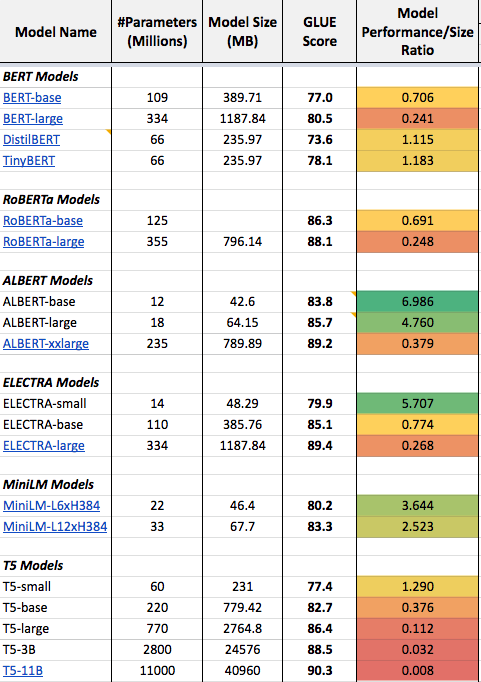Source: Language model comparision — Full evaluation here — Created by author

To provide more context the Model Performance/Size Ratio Scores are calculated as follows:

``Model Performance/Size Ratio = GLUE Score / Number of model parameters``

Described in words, the ratio is higher for a model if the model performs better on the GLUE benchmark with fewer parameters. Thus, the higher the number in the Model Performance/Size Ratio column the better.

### Transformer — ALBERT fine-tuning

A Transformer is a neural network architecture that uses a self-attention mechanism, allowing the model to focus on the relevant parts of a sentence to improve prediction qualities. The self-attention mechanism is able to connect all word tokens with each other at once, leading to the creation of long-term dependency understandings. As evaluated in the previous section, the ALBERT models do these most effectively among all the transformer language models available today.

### Load ALBERT tokenizer and pre-trained network

The first step to initiate the fine-tuning procedure is to load the ALBERT-large model from TensorflowHub which we will use as a base for the fine-tuning process.

Furthermore, we do the same and load the Albert tokenizer which converts the financial news sequences into sequences of tokens.

### Data input pipeline

The Albert tokenizer is used in a Data Generator function that creates a constant stream of batches to our ALBERT network. Within the Data Generator, the news sequences are being tokenized, in addition, the sentiment labels are hot encoded. Thus, the data input stream is set up properly and concluded.

### Create fine-tune transformer model

The second step is to define the model architecture of your fine-tuned ALBERT. In order to concentrate on the core, we simply parse the ALBERT-large model into a single layer (albert_layer) of our network. After this, we add a classification head with 2 Dense Layers, which have 64 and 3 (Number of classes) parameters, respectively. Subsequently, we compile the model with a Categorical Crossentropy Loss and an Adam optimizer with an adjusted learning rate.

### Fine-tune ALBERT

The last step is to call our create_model() function from before and initiate the training process.

## Results

The training process has a total number of 25 epochs. After the training, when looking at the confusion matrix we can see that our ALBERT transformer only assigns 6 records to the wrong classes. Thus, for the validation set our fine-tuned model achieves an accuracy of 97.46%.

The rest of the validation dataset metrics show similar impressive results. Precision and Recall both have a value above 97% and are very similar which means that rebalancing the class distribution via the reverse class weights worked successfully.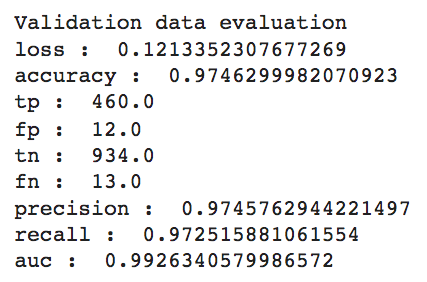Source: Fine-tune ALBERT, a metric overview of validation dataset— Created by author

## Conclusion

In conclusion, we trained a sentiment classifier for financial news articles which is close to achieving a perfect accuracy score. To achieve these results, we used a pre-trained ALBERT-large language model which has one of the best ratios between model performance and model size.